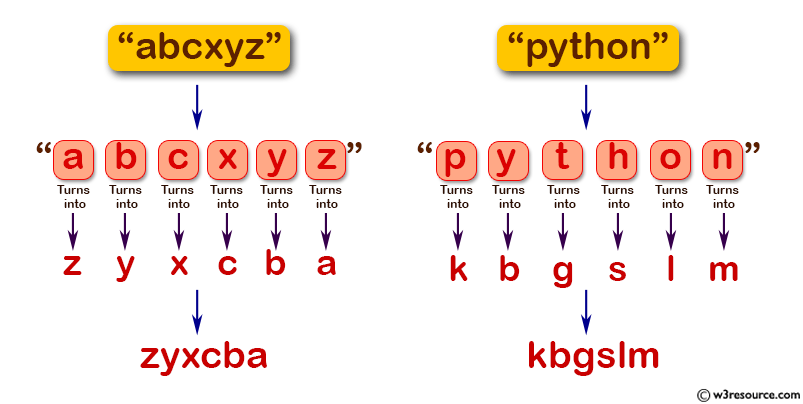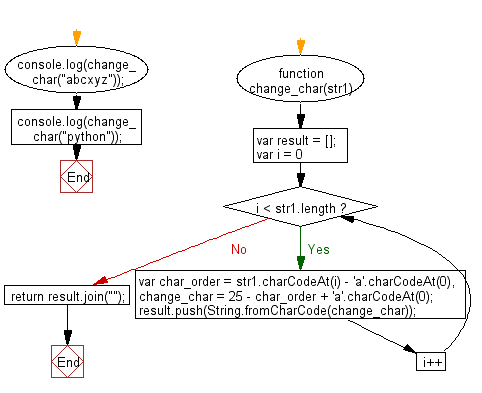# JavaScript: Change the characters (lower case) in a string where a turns into z, b turns into y, c turns into x, ..., n turns into m, m turns into n, ..., z turns into a

## JavaScript Basic: Exercise-134 with Solution

Write a JavaScript program to change the characters (lower case) in a string where a turns into z, b turns into y, c turns into x, ..., n turns into m, m turns into n, ..., z turns into a.

Pictorial Presentation:Sample Solution:

HTML Code:

``````<!DOCTYPE html>
<html>
<meta charset="utf-8">
<meta name="viewport" content="width=device-width">
<title>Change the characters (lower case) in a string where a turns into z, b turns into y, c turns into x, ..., n turns into m, m turns into n, ..., z turns into a</title>
<body>

</body>
</html>
```
```

JavaScript Code:

``````function change_char(str1) {
var result = [];
for (var i = 0; i < str1.length; i++)
{
var char_order = str1.charCodeAt(i) - 'a'.charCodeAt(0),
change_char = 25 - char_order + 'a'.charCodeAt(0);
result.push(String.fromCharCode(change_char));
}
return result.join("");
}
console.log(change_char("abcxyz"));
console.log(change_char("python"));
``````

Sample Output:

```zyxcba
kbgslm
```

Flowchart:ES6 Version:

``````function change_char(str1) {
const result = [];
for (let i = 0; i < str1.length; i++)
{
const char_order = str1.charCodeAt(i) - 'a'.charCodeAt(0);
const change_char = 25 - char_order + 'a'.charCodeAt(0);
result.push(String.fromCharCode(change_char));
}
return result.join("");
}
console.log(change_char("abcxyz"));
console.log(change_char("python"));
``````

Live Demo:

See the Pen javascript-basic-exercise-134 by w3resource (@w3resource) on CodePen.

Improve this sample solution and post your code through Disqus

What is the difficulty level of this exercise?

Test your Programming skills with w3resource's quiz.

﻿

## JavaScript: Tips of the Day

Checks if a string is an anagram of another string (case-insensitive, ignores spaces, punctuation and special characters)

Example:

```const isAnagram = (str1, str2) => {
const normalize = str =>
str
.toLowerCase()
.replace(/[^a-z0-9]/gi, '')
.split('')
.sort()
.join('');
return normalize(str1) === normalize(str2);
};
console.log(isAnagram('iceman', 'cinema')); // true
```

Output:

```true
```